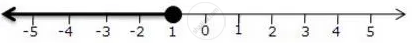Share

For Graph Given Write an Inequation Taking X as the Variable - ICSE Class 10 - Mathematics

ConceptLinear Inequations in One Unknown

Question

For graph given write an inequation taking x as the variableSolution

x <= -1, x in R

Is there an error in this question or solution?

APPEARS IN

Solution For Graph Given Write an Inequation Taking X as the Variable Concept: Linear Inequations in One Unknown.
S